HOME COURSES PREVIEW REVIEW ABOUT CONTACTTOLL-FREE INFO & ORDERING: M-F: 9am-5pm (PST): (877) RAPID-10

 24/7 Technical Support:

 Quick Search: Keywords:

 Rapid Learning Member Area: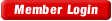Note: If you are a legacy user of chemistry24 members, please request a new login access to the premium server with your full name and old login email via vip@rapidlearningcenter.com

 Rapid Courses Catalog : Physics in 24 Hours Chemistry in 24 Hours Biology in 24 Hours Mathematics in 24 Hours

Weekly Physics Tips:
Want to become a top gun in your class? How about study less yet score high? Sign up this Physics Survival Weekly to learn how. Designed specifically for students who are taking physics, this free newsletter will show you how to survive and excel in class! Weekly topics include:
• How to Study Physics Effectively
• How to Read Physics Textbooks Easily
• How to Solve Physics Problems Systematically
• How to Score High on Physics Exams Strategically
• How to Master Physics Rapidly
Each week, you will receive study tips on the topics above and visual tutorial or study template to enhance your physics learning. Enter your name and email below to subscribe free:

 Your Name * Email *

 Physics Study Lounge These study sheets are for quick review on the subjects. Refer to our rapid courses for comprehensive review.     - Basic Skills in Physics     - Basic Math for Physics     - How to Solve Physics Problems     - Newton's Laws Study Guide     - ElectroMagnetism Quick Review     - Atomic Physics At-A-Glance     - Core Concepts in Nuclear Physics     - Special Relativity Overview

 Other Related Sites
 Note: For course links to launch, disable popup blockers or hold the ctrl key while clicking the link.Home » College Calculus

Algebra Review

 Topic Review on "Title": Algebra: Mathematics branch of mathematics that uses basic operations to solve expressions Linear equations: A linear equation is an equation involving only the sum or product of constants and the first power of a variable.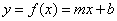where m is the slope and b is the y intercept is the general form of an equation in slope intercept form. The formula of a slope=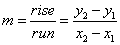where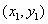and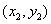are two points in ordered pair form. The general form of point slope form is: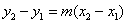where m is the slope,andis the two points in ordered pair form.   Polynomial equations: The quadratic formula equals: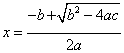where a, b and c are constants of a quadratic equation is of the form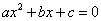. Factoring: Factoring is a process of dividing out a factor from a mathematical expression. Common factors technique is of the form ax+bx=x(a+b). Difference of squares: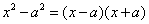. Difference of cubes: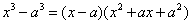. Rationalizing is the process of removing an irrational expression from the numerator of a fraction. Order and Inequalities:    Number line: An axis or ray usually horizontal on which real numbers are represented and ordered from left to right.    Absolute value: For a real number a, it is a if a is greater than or equal to zero or –a if a is less than zero. It is denoted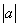. Conic sections: The general equation of a circle: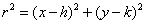. Where r is the radius of the circle and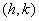is the center of the circle The general equation of an ellipse: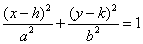. Natural logarithm: A log taken to base “e”, is approximately 2.7. Natural Log Function: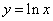. Exponential function: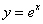.

Rapid Study Kit for "Title":
 Flash Movie Flash Game Flash Card Core Concept Tutorial Problem Solving Drill Review Cheat Sheet"Title" Tutorial Summary : This tutorial deals with the algebraic concepts needed to have a strong knowledge foundation for a Calculus course. Linear and quadratic equations are needed to be introduced in this Calculus course because they will be referred to in some Calculus topics. Specific properties of the basic algebraic concepts needed for a Calculus course are discussed in this tutorial. Important equations such as logarithmic and exponential equations are mentioned in this tutorial so a deeper understanding of them is developed which will be needed for some differentiation and integration ideals.

 Tutorial Features: Specific Tutorial Features: Linear equations and inequalities are introduced in this tutorial with the help of examples. The graphical representation of inequalities are covered in this tutorial along with their use with linear equations. Series Features: Concept map showing inter-connections of new concepts in this tutorial and those previously introduced. Definition slides introduce terms as they are needed. Visual representation of concepts Animated examples—worked out step by step A concise summary is given at the conclusion of the tutorial.

 "Title" Topic List: `The basic rules of AlgebraAlgebraic linear equations The graphical representation of a linear equation and its definition An equation and it slope intercept form Linear equation and its slopeEquations that have polynomials Quadratic equations and its solutions Higher polynomial equations Polynomial equations and their factoring Common polynomial factoring techniquesRationalizing of polynomial equationsOrder and Inequalities Number line and its graphical representation used in example problems How to solve simple linear inequalities?Absolute value How to solve absolute value inequalities?Inequalities that have polynomials How to solve polynomial inequalities?Conic Sections Ellipses The properties of ellipses Circles The properties of circlesExponentials and logarithms Exponentials and logarithmic functions and their definitions Graphs and applications of exponentials and logarithmic equations`

See all 24 lessons in college calculus, including concept tutorials, problem drills and cheat sheets:
Teach Yourself College Calculus Visually in 24 Hours